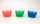# Axial section

Axial section of the cone is an equilateral triangle with area 208 dm2. Calculate the volume of the cone.

Result

V =  188.1 dm3

#### Solution:

$S = \pi \cdot \dfrac{a}{2}(\dfrac{a}{2}+a) = \dfrac{3}{4}\pi a^2 = 208 \ dm^2 \ \\ a = \sqrt{ \dfrac{4S}{3\pi}} = 9.4 \ dm \ \\ h = \sqrt {a^2-\dfrac{a^2}{2}} = 8.14 \ dm \ \\ \ \\ V = \dfrac{1}{3} \pi r^2 h = 188.1 \ dm^3$

Leave us a comment of this math problem and its solution (i.e. if it is still somewhat unclear...):Be the first to comment!#### Following knowledge from mathematics are needed to solve this word math problem:

Tip: Our volume units converter will help you with the conversion of volume units. Pythagorean theorem is the base for the right triangle calculator. See also our trigonometric triangle calculator.

## Next similar math problems:

1. Cone A2VSurface of cone in the plane is a circular arc with central angle of 126° and area 415 cm2. Calculate the volume of a cone.
2. CuboidCuboid with edge a=16 cm and body diagonal u=45 cm has volume V=11840 cm3. Calculate the length of the other edges.
3. Secret treasureScouts have a tent in the shape of a regular quadrilateral pyramid with a side of the base 4 m and a height of 3 m. Determine the radius r (and height h) of the container so that they can hide the largest possible treasure.
4. CubesOne cube is inscribed sphere and the other one described. Calculate difference of volumes of cubes, if the difference of surfaces in 257 mm2.
5. Space diagonalThe space diagonal of a cube is 129.91 mm. Find the lateral area, surface area and the volume of the cube.
6. Body diagonalCalculate the volume of a cuboid whose body diagonal u is equal to 6.1 cm. Rectangular base has dimensions of 3.2 cm and 2.4 cm
7. The cuboidThe cuboid is filled to the brim with water. The external dimensions are 95 cm, 120 cm, and 60 cm. The thickness of all walls and the bottom is 5 cm. How many liters of water fit into the cuboid?
8. Alien shipThe alien ship has the shape of a sphere with a radius of r = 3000m, and its crew needs the ship to carry the collected research material in a cuboid box with a square base. Determine the length of the base and (and height h) so that the box has the larges
9. Three glassesThree glasses of different colors have different volumes. Red 1.5 liter is filled from 2/5, blue 3/4 liter is filled from 1/3, and the third green 1.2 liter is empty. Pour green glass 1/4 of the contents from the red glass and 2/5 of the content from the b
10. TV transmitterThe volume of water in the rectangular swimming pool is 6998.4 hectoliters. The promotional leaflet states that if we wanted all the pool water to flow into a regular quadrangle with a base edge equal to the average depth of the pool, the prism would have.
11. Cube in a sphereThe cube is inscribed in a sphere with volume 9067 cm3. Determine the length of the edges of a cube.
12. Transforming cuboidCuboid with dimensions 6 cm, 10, and 11 cm is converted into a cube with the same volume. What is its edge length?
13. TerezaThe cube has area of base 256 mm2. Calculate the edge length, volume and area of its surface.
14. The cylinder 2Find the volume and the lateral area of a cylinder of height 12 inches and a base radius of 4 inches.
15. Rectangular cuboidThe rectangular cuboid has a surface area 5334 cm2, its dimensions are in the ratio 2:4:5. Find the volume of this rectangular cuboid.
16. Cylinder and its circumferenceIf the height of a cylinder is 4 times its circumference. What is the volume of the cylinder in terms of its circumference, c?
17. PoolThe swimming pool is 10 m wide and 8 m long and 153 cm deep. How many hectoliters of water is in it, if the water is 30 cm below its upper edge?